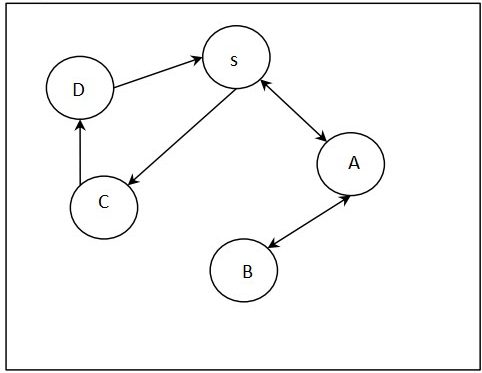# Finding the path from one vertex to rest using BFS in C++

C++Server Side ProgrammingProgramming

In this problem, we are given a directed graph represented as an adjacency list. Our task is to create a program for finding the path from one vertex to rest using BFS.

BFS(Breadth First Search) is an algorithm that traverses a graph in a breadthward motion and uses a queue to remember to get the next vertex to start a search, when a dead end occurs in any iteration.

Let's take an example to understand the problem,

InputOutput

S

A <= S

B <= A <= S

C <= S

D <= C <= S

## Solution Approach

To solve the problem, we will be performing the BFS search algorithm on each element of the graph. To perform the task, we will be creating a queue which will be storing the flag for visits to any node. Then, using a visited array we will be checking if the element is visited or not (binary values 0 and 1 denoting the visit).

Now, we will be solving the example step by step to understand the working of our solution,Starting from node S,

• We will directly visit node A.

• To reach node B, we will visit node A first then reach node B traversing node A.

• To reach node C, we will directly visit C from S.

• To reach node D, we will visit node C first and then node D.

## Example

Program to illustrate the working of our solution

#include <bits/stdc++.h>
using namespace std;
void printPath(vector<int> parent, int initial, int node){
while (initial != node){
cout<<node<<" <= ";
node = parent[node];
}
cout<<node<<endl;
}
void findPathBFS(vector<vector<int> > graphAdjList, int initial, int graphSize){
vector<int> parent(graphSize, 0);
vector<int> queue(graphSize, 0);
int front = -1, rear = -1;
vector<int> isVisited(graphSize, 0);
isVisited = 1;
parent = initial;
queue[++rear] = initial;
int k;
while (front != rear)
{
k = queue[++front];
if (isVisited[j] == 0){
queue[++rear] = j;
isVisited[j] = 1;
parent[j] = k;
}
}
}
for (k = 0; k < graphSize; k++)
printPath(parent, initial, k);
}
int main(){
int initial = 0;
cout<<"The Path from vertex '0' to all other vertex in the graph is : \n";
}
The Path from vertex '0' to all other vertex in the graph is :
4 <= 3 <= 0